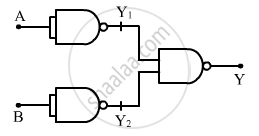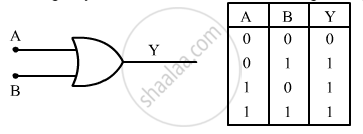Share

# Identify the Equivalent Gate Represented by the Circuit Shown in the Figure. Draw Its Logic Symbol and Write the Truth Table. - Physics

ConceptIntegrated Circuits

#### Question

Identify the equivalent gate represented by the circuit shown in the figure. Draw its logic symbol and write the truth table.This question doesn't have a solution currently. Please check again in a few days.

#### Notes

Truth table for the given circuit:

 A B Y1 Y2 Y 0 0 1 1 0 0 1 1 0 1 1 0 0 1 1 1 1 0 0 1

From the table, we can conclude that the given circuit represents an OR gate.
The logic symbol and truth table for an OR gate is given below:Is there an error in this question or solution?

#### APPEARS IN

Solution Identify the Equivalent Gate Represented by the Circuit Shown in the Figure. Draw Its Logic Symbol and Write the Truth Table. Concept: Integrated Circuits.
S